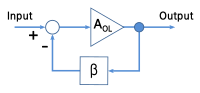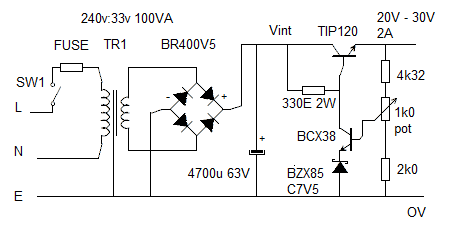# John Errington's tutorial on Power Supply Design

## Voltage regulation : performance analysis

### General AnalysisPower supply regulators are basically Negative Feedback Amplifiers. Negative feedback improves performance (gain stability, linearity, frequency response and step response) and reduces sensitivity to parameter variations (Hfe, Vbe etc).

Most importantly for our purposes, negative feedback reduces output resistance by a factor of 1/1+AB where A is the amplifier gain, and B the fraction fed back.

### Analysis Applied to RS2This is DE1 + RS2 with our calculated component values shown on the diagram.

TR1 (TIP120) is in an emitter follower configuration, with a voltage gain of very nearly unity;

TR2 (BCX38) is a comon emitter amplifier with a gain of -Rc / Re where Rc is R1(330) // Hfe Rload and Re is Rz.
For a BZX 85 series zener Rz is roughly the same as the zener voltage. ie for an 7.5V zener Rz = 7.5 ohms.

Suppose our load is drawing 2A at 20V then Rload = 10 ohms and Hfe * Rload = 2000 * 10 = 20k.

As this is very much bigger than R1 it can be ignored as 330//20k = 330

R1=330 ohm and Rz = 7.5 ohm so we have a voltage gain of 330/7.5 = 40.

The feedback fraction is just the ratio of voltages - when Vout = 30V this is 8.2 / 30 = 0.27

The effect of this is to reduce our calculated output resistance by a factor of 1/(1+ 40 * 0.27 ) = 1/10 approx

so we now have Rout = 0.1/10 = 0.01 ohm

### RS2 Summary

RS2 is a simple and very capable regulator for general use. It cannot be used to provide regulated voltages less than about 10V, but is able to be used to regulate high voltages by substituting a higher voltage zener diode, and transistors with suitable Vcbo (eg BU323Z, 250V)

The main factors limiting the performance of this supply now are:

• The voltage reference is Vz + Vbe which are both temperature dependent;
• The zener diode produces noise.
• The current through the reference still changes as the load or input voltage varies.

It would therefore not be suitable as a design for a highly stable precise or noise-free supply. We will improve this even further, at the expense of more components, in the next circuit.